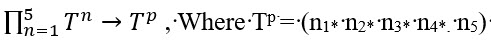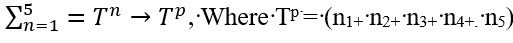Palindromic Mappings

Author: Robleh Wais 10/23/22

I have become interested in a topic that should be classified as part of number theory. This curiosity was sparked in me after observing the screen name of a youtuber which happens to be a palindromic sequence of letters: xylyxylyx.

After looking at them with intriguing interest for some time (I visit his YouTube site regularly), it occurred to me that this palindrome could be mapped to natural numbers (that is excluding zero). After that, the integers could be remapped to other palindromes via an operation like multiplication. From here, it was an obvious inference to suppose that an extension of the Palindromic Challenge could be created. Below is a description of the process. It is leading me to other perhaps more revealing areas to explore beyond what I am calling palindromic mappings.

The question is simple. But first we must construct the set.

If we take a N-tuple, where N=5, and use a seed S, where S=3, then we can generate an integral palindromic set for the 5-tuple, for S=(A,B,C) we have:

 A B C B A

0 is excluded, so we use the whole number subset of the integers. For the first set, where s= (1,2,3), we have the 5-tuple string

 1 2 3 2 1

If we multiply this 5-tuple string, the result is 12. If we set S= (4,5,6), then we have the 5-tuple string 4,5,6,5,4, If we multiple this 5-tuple string, the result is 2400.

Now, we can ask the question. Does any 5-tuple string, which uses increasing S subsets to generate palindromic sets, become a palindromic set? I've created an excel sheet that seeks to map these palindromic sets. But to do this, a few more operations have to be included. Please note this is related to, but not the same as, the Palindrome Problem:

Palindrome Conjecture

This problem says given an integer x= n (where n is number of digits) and xr is the reverse of x =n digits. F(x)= x+ xr . Check if F(x) is a palindrome. If it is not, iterate the process until a palindrome is the result.

Palindrome Multiplicative Mapping Conjecture

My problem is different. I want to know if a palindrome can produce another palindrome by mapping them with the operation * (multiplication).

So, to state the conjecture in formal language. We have the following:

Given the initial set S=1,2,3. S can generate a 5-tuple set Tn and it is palindromic, then for n=1 to 5 of T does the following construct generate another TpMultiplicative Palindrome Conjecture

Of course, if this can be proved for a 5-tuple set, I would like to expand the proof to be any N-tuple set of palindromes.

Likewise, all that remains to be defined mathematically is what is a palindrome, right? I am using the definition found on Wikipedia:

Interestingly, if we look for the same thing but use addition as the operation that maps these sets, there are a significant subset of palindromes that seem to occur and may be a regular subset of the superset, which would imply that all the theorems applicable to group theory hold for these bijective, isomorphic mappings.

To restate the conjecture for addition we have:Additive Palindrome Conjecture

,

This formulation does yield palindromic sets. Below is an excerpt of some of them.

 A B C B A Tp 1 2 3 2 1 9 8 9 10 9 8 44 19 20 21 20 19 99 80 81 82 81 80 404 82 83 84 83 82 414 84 85 86 85 84 424 86 87 88 87 86 434 88 89 90 89 88 444 90 91 92 91 90 454 92 93 94 93 92 464 94 95 96 95 94 474 96 97 98 97 96 484 98 99 100 99 98 494 181 182 183 182 181 909 183 184 185 184 183 919 185 186 187 186 185 929 187 188 189 188 187 939 189 190 191 190 189 949 191 192 193 192 191 959 193 194 195 194 193 969 195 196 197 196 195 979 197 198 199 198 197 989 199 200 201 200 199 999 800 801 802 801 800 4004 822 823 824 823 822 4114 844 845 846 845 844 4224 866 867 868 867 866 4334 888 889 890 889 888 4444 910 911 912 911 910 4554 932 933 934 933 932 4664 954 955 956 955 954 4774 976 977 978 977 976 4884 998 999 1000 999 998 4994 1801 1802 1803 1802 1801 9009

What this table shows is that cardinal palindromes under addition do form ordinal palindromes. They seem to occur in intervals. Further analysis will be needed to determine if this algebraic mapping does in fact result in a continuous set relation. I have begun to see a recurrent pattern already. Is there any reason why adding together numbers that are palindromic by their values should become palindromic by their order? I don't see any intrinsic reason this should be. It seems strangely related to Fibonacci's Theorem.